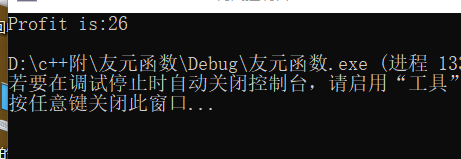# c++第三次作业：类的友元

## C++第三次作业：类的友元

1、友元的关系提供了不同类或对象的成员函数之间、类的成员函数与一般函数之间进行数据共享的机制。通俗的说友元关系就是一个类主动声明其他函数是他的朋友，可以使其获得特殊访问权利。
2、在友元函数中，是在类中用关键词friend修饰的非成员函数。虽然它不是本类的成员函数，但是在它的函数体可以通过对象名访问类的私有和保护成员。

#include"oo.h"
using namespace std;
class A {
public:
A(int x = 0, int y = 0) :price(x), cost(y) {};
friend int profit(A& a);
private:
int price, cost;
};
int profit(A& a) {
return (a.price-a.cost);
}
int main() {
A pa(58, 32);
cout << "Profit is:";
cout << profit(pa) << endl;
return 0;
}3、同友元函数一样，一个类可以将另一个类声明为友元类。若A类为B类的友元类，则A类的所有成员函数都是B类的友元函数，都可以访问B类的私有成员和保护成员。

class A {
public:
A(int x = 0, int y = 0) :price(x), cost(y) {};
int getp() { return price; }
int getc() { return cost; }
friend class B;
private:
int price, cost;
};
class B {
public:
void show();
private:
A a;
};
void B::show() {
cout << "price is:" << a.getp()<< endl;
cout << "cost is:" << a.getc() << endl;
}
int main() {
B mb;
mb.show();
return 0;
}

1、友元的关系是不能传递的；
2、友元关系是单向的；
3、友元关系是不被继承的。

posted @ 2019-09-25 18:35  咿呀琳琳  阅读(199)  评论(0编辑  收藏  举报